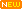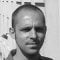Learn about power engineering and HV/MV/LV substations. Study specialized technical articles, electrical guides, and papers.

# Spreadsheet for Transformer Losses Calculation

## Transformer Losses

Transformers have two major components that drive losses: the core and the coils. The typical core is an assembly of laminated steel, and core losses are mostly related to magnetizing (energizing) the core.

These losses, also known as no-load losses, are present all the time the transformer is powered on – regardless of whether there is any load or not.

Core losses are roughly constant from no-load to full-load when feeding linear loads. They represent a continuous cost, 24 hours/day, for the 25-year or more life of the transformer.

• HV Full load current = VA / (1.732 · Volt)
• LV Full load current = VA / (1.732 · Volt)
• HV Side I2R losses = I²R · 1.5
• LV Side I²R losses = I²R · 0.5 · 3
• Total I² R lossses at Amb. temp = Hv losses + Lv losses
• Total Stray losses at Amb. temp = Measured losses – I²R losses
• I²R lossses at 75° C temp = ((225 + 75) · losses) / (225 + Amb. temp) .
• Stray losses at 75° C temp=((225 + Amb. temp)(Stray losses at Amb. temp)) / 300
• Total Full load losses at 75° C = I²R losses at 75° C + Stray losses at 75° C
• Total Impedance at amb. temp = (Imp. voltage · 1.732) / Full load current
• Total Resistance at amb. temp = I²R losses / I²
• Total Reactance (X) = SQRT (Impedance² – Resistance²)
• Resistance at 75° C = (300 · resistance at amb ) / (225 + Amb. temp)
• Impedance at 75° C = SQRT (R² at 75° C + X²)
• Percentage Impedance = (Z at 75° C · I · 100)/V1
• Percentage Resistance = ( R 75° C · I · 100)/V1
• Percentage Reactance = (X · I · 100) / V
• Regulation at Unity P.F. = (%R cosø + %Xsinø)
• Regulation at 0.8 P.F. = (%R cosø + %Xsinø) + 1/200(%R sinø – %Xcosø)2

#### Efficiency at Unity P.F

• At 125 % of Transformer Loading = (kVA · 1.25 · 100)/((kVA · 1.25)+(I²R losses · 1.25²)+(No Load Losses))

#### Efficiency at 0.8 P.F

• At 125 % of Transformer Loading = (kVA · 1.25 · P.F. · 100)/((kVA · P.F. · 1.25)+(I²R losses · 1.25²)+(No Load Losses))

## Contribution to transformer losses

This difference substantially impacts actual losses incurred. Unfortunately, there is a small body of field data available, driven by the factors such as a lack of awareness of the cost of the losses, and the cost of gathering detailed data from a reasonable number of individual transformers.

Several variables contribute to transformer losses, the most important of which include load level, load profile, and core and coil construction.

Since there are a wide variety of transformers on the market serving different purposes, and available from different manufacturers, actual losses incurred in the field will vary substantially from installation to installation.

 Software: Spreadsheet for Transformer Losses Calculation Version: 10.06.2013Developer: Jignesh Parmar Size: 36 Kb Price: Free Download: Right here | Video Courses | Membership | Download Updates

1.Shafique
Jun 26, 2020

Sir, coul you explain temperature gradient calculation of HV and LV winding , if temperature rise 40/50

2.Rohit Saini
Dec 12, 2019

3.Nandini Basavarajappa Doddaveerappla
Oct 15, 2019

Tell me how to get transformer copper loss
I have no load losses n total power loss

4.RAJESHKUMAR
Aug 28, 2019

Dear sir,
Dry type and oil type transformer losses how to calculate.

5.FLOYD
Jan 19, 2019

specs of hi pot tester from 10 to 35kv?

6.S.M.Tayyab
Nov 12, 2018

How can I calculate no load losses and load losses of power trafo when I have total losses of trafo available only?

7.Duaa Alqaruty
Sep 20, 2018

Great work, keep it up ^_^

8.Carcky
Jul 25, 2018

On this formula: Total Stray losses at Amb. temp = Measured losses – I²R losses, if I measured V= 384, I= 20 and W= 1015, for a 24MVA, 231200V (on tap 1), how do I relate the measured power into the equivalent input power which I can use in the formula?

9.sam oketch
Apr 18, 2018

The spreadsheet for transformer losses calculations is a good work

10.mani
Feb 04, 2018

how do find out the value of resistance in hv and lv side

11.Genaro Lucas Jr
Nov 25, 2017

please review cell H33, i think efficiency should not be highest at 125%

12.Oct 01, 2016

Dear all,

How can I get the value 1.5 in ” HV Side I2R losses = I²R · 1.5″ and 0.5*3 in
” LV Side I²R losses = I²R · 0.5 · 3″ .

13.Oct 01, 2016

Dear all,

What are the values 1.5 in HV and 0.5*0.3 and LV ?

HV Side I2R losses = I²R · 1.5
LV Side I²R losses = I²R · 0.5 · 3

14.venjateswarlu
Jul 30, 2015

Dear sir,
For finding i^2R losses on hv side =I^2R.1.5
What does 1.5 means

•Oct 17, 2016

Dear Venjateswar,

Did you find any answer for this question ?

Best regards,

15.hafizah
Jun 09, 2015

Hello!

what is V1 and V for the percentage impedance and reactance?

Thanks!

•John Njuguna
Nov 16, 2017

The V1 is the voltage that, applied to the HV side of the Tx whose voltage rating is V, shall circulate the rated current on the LV side of the Tx with it’s terminal short circuited. This voltage is expressed as a %age.
Z%=(V1/V)*100.

16.hafizah
Jun 09, 2015

Is the measured loss a physically measured value? Is there a way of estimating this number based on the transformer size?

17.Wyn
Feb 12, 2015

Edvard / Jignesh
well done gents this is practical and useful I like it!.
Here is a suggestion for you ready reckoner spread sheets for estimating:
overhead power lines e.g. 415V AC distance covered number of bends and calculate the number of posts, cross arms, insulators, conductor sizes, tension, weight of the conductors….
same for 22kV or 33kv or underground with approximate schedules of rates so I can enter local steel costs ideal pole spacing typical sag heights….

18.deepak
Jan 03, 2015

can you provide spreadsheet for transformer losses calculation of 1000kva

•Edvard
Jan 03, 2015

Can’t you use this spreadsheet? Just edit field rated power in kVA.

19.Mario Tovar
Oct 13, 2014

How can we convert this losses to thermal losses?

20.•